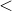## 6.3.2 Using lists as buffers

The following example demonstrates the use of a list as a buffer in which the first item added to the stack is the first one to be popped:

pyxplot> myList = []
pyxplot> for (i=1; i12; i++)
pyxplot>  {
pyxplot>   if prime(i) { call myList.append(i) ; }
pyxplot>  }
pyxplot> while (myList) { print myList.pop(0) ; }
2
3
5
7
11

The function prime(x) returns true if x is a prime number, and false otherwise. In the final line, we make use of the fact that a list tests true if it contains any items, or false if it is empty.